Online Tution   »   Class 10   »   CBSE Class 10 Maths MCQ Questions...

# CBSE Class 10 Maths MCQ Questions With Answers

CBSE Class 10 Maths (Standard and Basic) Important MCQ Questions With Answers: It’s exam season, and we all want to perform our best, so why not combine our best efforts with some cunning work? We will surely receive the highest possible score as a result of this.
Brush up on some good CBSE Class 10 Maths (Standard and Basic) Important MCQ Questions With Answers from Class 10 Math topics to help you prepare for the Boards exam and increase your chances of achieving 90% or above. This page has MCQ questions for all chapters of mathematics. Students taking the CBSE Class 10 Mathematics Board Exams are encouraged to practise as many questions as they can, and these MCQs for Mathematics class 10 have been created by the professionals themselves!

Class 10 Maths Sample Paper 2023 (Standard and Basic)

Class 10 Maths MCQ Question with Answers

Class 10 Maths Important Question with Answers

Class 10 Maths Previous Year Question Papers

We have not only provided answers to the Class 10 Maths MCQs but also explanations.

## Class 10 Maths MCQ (Standard) Important Questions With Answers

Q1. The shape of an ice-cream cone is a combination of:

(a) Sphere + cylinder

(b) Sphere + cone

(c) Hemisphere + cylinder

(d) Hemisphere + cone

Explanation: The shape of an ice-cream cone is a combination of a hemisphere and a cone.

Q2. If a cone is cut parallel to the base of it by a plane in two parts, then the shape of the top of the cone will be a:

(a) Sphere

(b) Cube

(c) Cone itself

(d) Cylinder

Explanation: If we cut a cone into two parts parallel to the base, then the shape of the upper part remains the same.

Q3. If we cut a cone in two parts by a plane parallel to the base, then the bottom part left over is the:

(a) Cone

(b) Frustum of cone

(c) Sphere

(d) Cylinder

Q4. If r is the radius of the sphere, then the surface area of the sphere is given by;

(a) 4 π r2

(b) 2 π r2

(c) π r2

(d) 4/3 π r2

Explanation: If r is the radius of the sphere, then the surface area of the sphere is given by 4 π r2.

Q5. If we change the shape of an object from a sphere to a cylinder, then the volume of cylinder will

(a) Increase

(b) Decrease

(c) Remains unchanged

(d) Doubles

Explanation: If we change the shape of a three-dimensional object, the volume of the new shape will be same.

Q6. Fifteen solid spheres are made by melting a solid metallic cone of base diameter 2cm and height 15cm. The radius of each sphere is:

(a) ½

(b) ¼

(c) 1/3√2

(d) 1/3√4

Explanation: Volume of 15 spheres = Volume of a cone
15 x (4/3) π r3 = ⅓ πr2h

5×4 π r3 = ⅓ π 12(15)

20r3 = 5

r3 = 5/20 = ¼

r = 1/3√4

Q7. The radius of the top and bottom of a bucket of slant height 35 cm are 25 cm and 8 cm. The curved surface of the bucket is:

(a) 4000 sq.cm

(b) 3500 sq.cm

(c) 3630 sq.cm

(d) 3750 sq.cm

Explanation: Curved surface of bucket = π(R1 + R2) x slant height (l)

Curved Surface = (22/7) x (25 + 8) x 35

CSA = 22 x 33 x 5 = 3630 sq.cm.

Q8. If a cylinder is covered by two hemispheres shaped lid of equal shape, then the total curved surface area of the new object will be

(a) 4πrh + 2πr2

(b) 4πrh – 2πr2

(c) 2πrh + 4πr2

(d) 2πrh + 4πr

Explanation: Curved surface area of cylinder = 2πrh

The curved surface area of hemisphere = 2πr2

Here, we have two hemispheres.

So, total curved surface area = 2πrh + 2(2πr2) = 2πrh + 4πr2

Q9. A tank is made of the shape of a cylinder with a hemispherical depression at one end. The height of the cylinder is 1.45 m and radius is 30 cm. The total surface area of the tank is:

(a) 30 m

(b) 3.3 m

(c) 30.3 m

(d) 3300 m

Explanation: Total surface area of tank = CSA of cylinder + CSA of hemisphere

= 2πrh + 2πr2= 2π r(h + r)

= 2 x 22/7 x 30(145 + 30) cm2

=33000 cm2

= 3.3 m2

Q10. If we join two hemispheres of same radius along their bases, then we get a;

(a) Cone

(b) Cylinder

(c) Sphere

(d) Cuboid

If we join two hemispheres of same radius along their bases, then we get a Sphere.

Q11. The median of the data 13, 15, 16, 17, 19, 20 is:

(a) 30/2

(b) 31/2

(c) 33/2

(d) 35/2

Explanation: For the given data, there are two middle terms, 16 and 17.

Hence, median = (16 + 17)/2 = 33/2

Q12. If the mean of first n natural numbers is 3n/5, then the value of n is:

(a) 3

(b) 4

(c) 5

(d) 6

Explanation: Sum of natural numbers = n(n + 1)/2

Given, mean = 3n/5

Mean = sum of natural numbers/n

3n/5 = n(n + 1)/2n

3n/5 = (n + 1)/2

6n = 5n + 5

n = 5

Q13. If AM of a, a+3, a+6, a+9 and a+12 is 10, then a is equal to;

(a) 1

(b) 2

(c) 3

(d) 4

Explanation: Mean of AM = 10

(a + a + 3 + a + 6 + a + 9 + a + 12)/5 = 10

5a + 30 = 50

5a = 20

a = 4

Q14. The class interval of a given observation is 10 to 15, then the class mark for this interval will be:

(a) 11.5

(b) 12.5

(c) 12

(d) 14

Explanation: Class mark = (Upper limit + Lower limit)/2

= (15 + 10)/2

= 25/2

= 12.5

Q15. If the sum of frequencies is 24, then the value of x in the observation: x, 5,6,1,2, will be;

(a) 4

(b) 6

(c) 8

(d) 10

Explanation:

Given,

∑fi = 24

∑fi = x + 5 + 6 + 1 + 2 = 14 + x

24 = 14 + x

x = 24 – 14 = 10

Q16. The distance between two parallel tangents of acircle of radius 4 cm is
(a) 2 cm
(b) 4 cm
(c) 6 cm
(d) 8 cm

Explanation: Here radius, r = 4 cm
Required distance,
AB = OA + OB
= r + r = 2r = 2×4 = 8 cm

Q17. In the given figure, if ZRPS = 25°, the value of ZROS is

(a) 135°
(b) 145°
(c) 165°
(d) 155°

Explanation: Reason: Since OR ⊥ PR and OS ⊥ PS
∴ ∠ORP = ∠OSP = 90°
In □ ORPS, ∠ROS + ∠ORP + ∠RPS + ∠OSP = 360°
∠ROS + 90° + 25° + 90° = 360°
∠ROS = 360° – 205° = 155°

Q18. A tangent is drawn from a point at a distance of 17 cm of circle C(0, r) of radius 8 cm. The length of its tangent is
(a) 5 cm
(b) 9 cm
(c) 15 cm
(d) 23 cm

Explanation: In rt ∆OAP, AP² + OA² = OP²
⇒ AP² + (8)² = (17)² => AP² + 64 = 289
⇒ AP² = 289 – 64 = 225
∴ AP = √225 = 15 cm

Q19. The length of tangents drawn from an external point to the circle
(a) are equal
(b) are not equal
(c) sometimes are equal
(d) are not defined

Explanation: Since the length of tangents drawn from an external point to a circle are equal.

Q20. Number of tangents drawn at a point of the , circle is/are
(a) one
(b) two
(c) none
(d) infinite

Explanation: There is only one tangent at a point of the circle.

Q21. The tangents drawn at the extremities of the diameter of a circle are
(a) perpendicular
(b) parallel
(c) equal
(d) none of these

Explaination:Reason: Since OP ⊥ AB and OQ ⊥ CD
∴ Z1 = 90° and Z2 = 90°
⇒ ∠1 = Z2, which are alternate angles.
∴ AB || CD

Q22. Tangents from an external point to a circle are
(a) equal
(b) not equal
(c) parallel
(d) perpendicular

Explanation: Reason: Tangents from external points to a circle are equal.

Q23. The length of a tangent drawn from a point at a distance of 10 cm of circle is 8 cm. The radius of the circle is
(a) 4 cm
(b) 5 cm
(c) 6 cm
(d) 7 cm

Explaination: In rt. AOAP, we have
OA² + AP² = OP²
⇒ OA² + (8)² = (10)2
⇒ OA² + 64 = 100
⇒ OA² = 100 – 64 = 36
∴ OA = √36 = 6 cm

Q24. To construct a triangle similar to a given ΔPQR with its sides 5/8 of the similar sides of ΔPQR, draw a ray QX such that ∠QRX is an acute angle and X lies on the opposite side of P with respect to QR. Then locate points Q1, Q2, Q3, … on QX at equal distances, and the next step is to join:

(a) Q10 to C

(b) Q3 to C

(c) Q8 to C

(d) Q4 to C

Explanation: Here we locate points Q1, Q2, Q3, Q4, Q5, Q6, Q7 and Q8 on QX at equal distances and in the next step join the last point Q8 to R.

Q25. To construct a triangle similar to a given ΔPQR with its sides, 9/5 of the corresponding sides of ΔPQR draw a ray QX such that ∠QRX is an acute angle and X is on the opposite side of P with respect to QR. The minimum number of points to be located at equal distances on ray QX is:

(a) 5

(b) 9

(c) 10

(d) 14

Explanation: To draw a triangle similar to a given triangle with its sides m/n of the similar sides of a given triangle, the minimum number of points to be located at an equal distance is equal to m or n, whichever is greater.

Here, m/n = 9/5

9 > 5, therefore the minimum number of points to be located is 9.

Q26. To construct a pair of tangents to a circle at an angle of 60° to each other, it is needed to draw tangents at endpoints of those two radii of the circle, the angle between them should be:

(a) 100°

(b) 90°

(c) 180°

(d) 120°

Explanation: The angle between the two radii should be 120° because the figure produced by the intersection point of pair of tangents and the two endpoints of those two radii and the centre of the circle, is a quadrilateral. Hence, the sum of the opposite angles should be 180°.

Q27. To divide a line segment PQ in the ratio m : n, where m and n are two positive integers, draw a ray PX so that ∠PQX is an acute angle and then mark points on ray PX at equal distances such that the minimum number of these points is:

(a) m + n

(b) m – n

(c) m + n – 1

(d) Greater of m and n

To divide a line segment PQ in the ratio m : n, where m and n are two positive integers, draw a ray PX so that ∠PQX is an acute angle and then mark points on ray PX at equal distances such that the minimum number of these points is m + n.

Q28. To draw a pair of tangents to a circle which are inclined to each other at an angle of 45°, it is required to draw tangents at the endpoints of those two radii of the circle, the angle between which is:

(a) 135°

(b) 155°

(c) 160°

(d) 120°

To draw a pair of tangents to a circle which are inclined to each other at an angle of 45°, it is required to draw tangents at the endpoints of those two radii of the circle, the angle between which is 135°.

Q29. A pair of tangents can be constructed from a point P to a circle of radius 3.5 cm situated at a distance of ___________ from the centre.

(a) 3.5 cm

(b) 2.5 cm

(c) 5 cm

(d) 2 cm

Explanation: The pair of tangents can be drawn from an external point only, so its distance from the centre must be greater than the radius. Since only 5cm is greater than the radius of 3.5 cm. So the tangents can be drawn from the point situated at a distance of 5 cm from the centre.

Q30. To construct a triangle ABC and then a triangle similar to it whose sides are 2/3 of the corresponding sides of the first triangle. A ray AX is drawn where multiple points at equal distances are located. The last point to which point B will meet the ray AX will be:

(a) A1

(b) A2

(c) A3

(d) A4

Explanation: The greater of 2 or 3 will be the maximum number of points. Hence, the last point will be A3.

Q31. To construct a triangle similar to a given ΔPQR with its sides 3/7 of the similar sides of ΔPQR, draw a ray QX such that ∠QRX is an acute angle and X lies on the opposite side of P with respect to QR. Then locate points Q1, Q2, Q3, … on QX at equal distances, and the next step is to join:

(a) Q10 to C

(b) Q3 to C

(c) Q7 to C

(d) Q4 to C

(a) two distinct real roots
(b) two equal real roots
(c) no real roots
(d) more than 2 real roots

Q33. The real roots of the equation  are
(a) 1,8
(b) 1,6
(c)1,12
(d)1,4

Q34. (-2,-4) has
(a) four real roots
(b) two real roots
(c) no real roots
(d) one real root

Q35. The equation , where  is real, always has roots that are
(a) Equal
(b) Equal in magnitude but opposite in sign
(c) Irrational
(d) Real

Answer: (b) Equal in magnitude but opposite in sign

Q36. The condition for one root of the quadratic equation  to be twice the other, is
(a)0
(b)1
(c)2

(a) two distinet real roots
(b) two equal real roots
(c) no real roots
(d) more than 2 real roots

Answer: (b) two equal real roots

(a) two distinct real roots
(b) two equal real roots
(c) no real roots
(d) more than 2 real roots

Answer: (a) two distinct real roots

Q39. The common difference of the AP  , is
(a) 1
(b)2
(c)-1
(d)0

Q40. The value of  for which  and  are the three consecutive terms of an , is
(a) 6
(b)12
(c) 18

(d)24

Q41. The first term of  is  and the common difference is , then its 10 th term is
(a)1000
(b)100
(c)10
(d)12

Q42. In an AP, if  and , then  is equal to
(a) 6
(b) 7
(c) 20
(d) 28

Q43. In an AP, if  and , then  will be
(a) 0
(b)1
(c)-1
(d)-2

Q44. The 11th term of an AP  is
(a)10
(b) 20
(c)15
(d) 30

Q45. In an AP, if  and , then  will be
(a) 0
(b)2
(c)1
(d)-1

Q46. Which term of an AP,  is
(a) 9 th
(b) 10th
(c)14th
(d)8th

Q47. If the common difference of an AP is 5 , then what is
(a) 5
(b) 20
(c) 25
(d) 30

## CBSE Class 10 Maths (Basic) Important MCQ Questions

Time Allowed: 90 minutes

Maximum Marks: 40

General Instructions:

1. You have 05 Minutes to complete the test.
2. The test contains a total of 10 Questions for 10 Marks.
3. There is only one correct answer to each question. Click on the most appropriate option to mark it as your answer.
4. There is 1/4 penalty for each wrong answer
5. You can change your answer by clicking on some other option.
6. You can unmark your answer by clicking on the “Clear Response” button.
7. A Number list of all questions appears at the right-hand side of the screen. You can access the questions in any order within a section or across sections by clicking on the question number given on the number list.
8. You can use rough sheets while taking the test. Do not use calculators, log tables, dictionaries, or any other printed/online reference material during the test.

1. Do not click the button “Submit test” before completing the test. A test once submitted cannot be resumed.

SECTION A

This section contains 13 MCQS with overall choice to attempt all questions.

Q is divisible by 8 , if  is
(a) an integer
(b) a natural number
(c) an odd integer
(d) an even integer

Q2. When  is divided by 17 the remainder would be
(a) 1
(b) 16
(c) 14
(d) None of these

Q3. If every positive even integer is of the form , then every positive odd integer is of the form ……… where  is some integer.
(a) 2q+1
(b) 2q-1
(c) 2q
(d) None of these

Q4. The exponent of 2 in the prime factorisation of 144 , is
(a) 2
(b) 4
(c) 6
(d) 8

Q5. , etc. are what numbers.
(a) rational
(b) integer
(c) irrational
(d) whole numbers

Q6. Every point on the number line corresponds to a number is
(a) imaginary
(b) real
(c) point
(d) rational

Q8. Find the condition that zeroes of polynomial  are reciprocal of each other.
(a) c=b
(b)c=x
(c) c=a
(d) a=c

Q9. Find the value of  if  is a zero of the polynomial

(a) 2
(b)-2
(c) 4
(d) 1

Q10. Find the values of  and , if they are the zeroes of polynomial

(a) 2
(b)0
(c) 4
(d) 1

SECTION A

S1.  (Ans) (c)

S2. (Ans) (a)

S3. (Ans) (a)

S4. (Ans) (b)

S5. (Ans) (c)

S6. (Ans) (b)

S7. (Ans) (a)

S8. (Ans) (c)

S9. (Ans) (b)

S10. (Ans) (d)

Related Articles:
Class 10 SST MCQs
Class 10 Science MCQs
Class 10 English MCQs

## Class 10 Term 1 Maths (Standard and Basic) Important MCQ QNA

### What is Z in real numbers?

Z stands for integers in real numbers.

### What is P in real numbers?

P stands for irrational numbers in real numbers.

### Which is more accurate: right math or maths?

In the United States and Canada, the term math is favoured, but Maths is favoured in the United Kingdom, Ireland, Australia, and other English-speaking countries.

### What are the four types of mathematics?

The four main branches of mathematics are Algebra, Geometry, Calculus, and Statistics & Probability.

### Who is considered as the “Father of Mathematics”?

The Greek mathematician Archimedes is recognised as the “Father of Mathematics.”

Sharing is caring!

## FAQs

### What is Z in real numbers?

Z stands for integers in real numbers.

### What is P in actual numbers?

P stands for irrational numbers in real numbers.

### Which is more accurate: right math or maths?

In the United States and Canada, the term math is favoured, but Maths is favoured in the United Kingdom, Ireland, Australia, and other English-speaking countries.

### What are the four types of mathematics?

The four main branches of mathematics are Algebra, Geometry, Calculus, and Statistics & Probability.

### Who is considered as the "Father of Mathematics"?

The Greek mathematician Archimedes is recognised as the "Father of Mathematics."

Thank You, Your details have been submitted we will get back to you.

### TOPICS:

•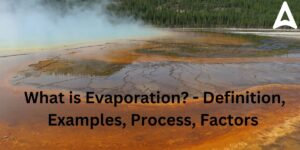Evaporation - Definition, Examples, Proc...
•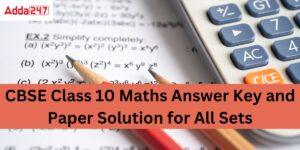Class 10 Maths Answer Key 2023, Set 1,2,...
•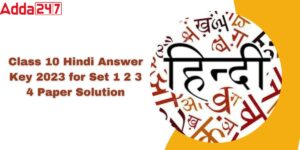Hindi Answer key 2023 Class 10 CBSE, Set...
•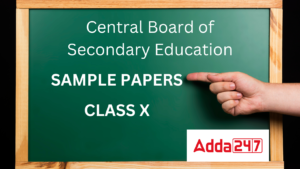Maths Sample Paper Class 10 2023 with So...
•Maths Sample Paper Class 12 2023 with CB...
•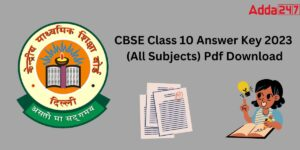CBSE Class 10 Answer Key 2023, All 10th ...